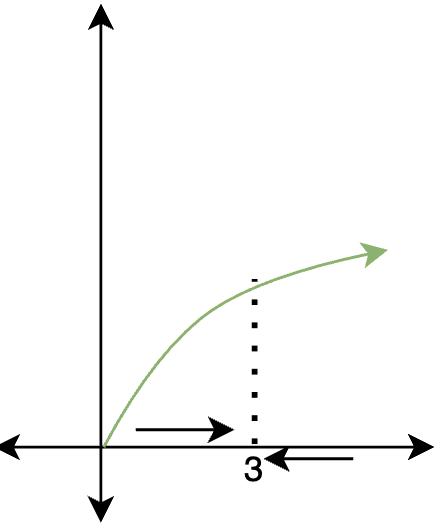GeeksforGeeks App
Open AppBrowser
Continue

# Limits by Direct Substitution

Limits are building blocks of calculus. They are the values that a function seems to be taking on while we reach a particular point. They help is calculating the rate of change of the functions. The concept of derivatives has been defined with limits. They also help us define the concepts of continuity and differentiability. So, it becomes essential to understand their intuition and different methods to work out the limits for the different categories of functions.

### Limits

Geometrically speaking, the limit of a function at a particular point can be easily estimated through its graph. For example, in the graph given below, at x = 3. The function seems to be taking on the value of 1. In this case, it does not matter from which side we approach — the left-hand side and right-hand side.For the function f(x), the limit at x = a is denoted by,### Limits using Direct Substitution

The substitution rule for calculating limits is a method of finding limits, by simply substituting the value of x with the point at which we want to calculate the limit. Consider a function f(x), the goal is to find the limit of the function at x = a. In this method, x is simply substituted with “a” in the expression for the function f(x).Let’s look at this method through an example,

Considering f(x) = x2Often it is possible to calculate the limits for the function with this rule, to state this formally.

If f(x) is an expression built from the polynomials, roots, absolute values, exponential, logarithms, trigonometric functions, and/or inverse trigonometric functions by using composition of functions and the operations such as x, +, -, / then for any a for which f(a) is defined,Undefined limits by direct substitution

There are certain limits which cannot be calculated by this method. For example, consider a function f(x) =, calculate the limit for this function at x = 1.This limit is undefined. In such cases, the direct substitution method.

Limits of Trigonometric Function

Direct substitution can sometimes be used to calculate the limits for functions involving trigonometric functions. For example, let’s say we have a function f(x) and we want to calculate the limits for that function at x = 0.  Let’s see this with an example.

Example: Calculate thef(x) = sin(x) + sin(x)cos(x)

Solution:⇒⇒0 × (1 + 1)

⇒ 0

Limits of Piecewise Function

When working with piecewise functions, the substitution rule generally does not work at the places where the definition of the function is changing. It is used in a little modified way for those functions. Let’s work out a sample problem to further understand this,

Example: Calculate the value ofSolution:

At x = 1, the function definition is changing. So it is not advised to directly apply the rule. In such functions, one should look for the limit from both sides.

Left-hand Side Limit⇒1

Right-hand Side Limit⇒0

In this case, limits from both sides are different.

Let’s see some problems on these concepts

### Sample Problems

Question 1: Calculate thef(x) = x2 + x + 1

Solution:⇒⇒ 1

Question 2: Calculate thef(x) =Solution:⇒ \⇒Question 3: Calculate the value ofSolution:

At x = 1, the function definition is changing. So it is not advised to directly apply the rule. In such functions, one should look for the limit from both sides.

Left-hand Side LimitRight-hand Side LimitIn this case, limits from both sides are different.

Question 4: Calculate thef(x) =Solution:⇒ \⇒⇒ e + 2

Question 5: Calculate thef(x) =Solution:⇒ \⇒⇒ esin(1) + 2

Question 6: Calculate theusing substitution rule.

f(x) =Solution:⇒ \This is 0/0 form, so the limit is undefined. The substitution rule cannot be used here.

Question 7: Calculate the value ofSolution:

At x = 1, the function definition is changing. So it is not advised to directly apply the rule. In such functions, one should look for the limit from both sides.

Left-hand Side LimitRight-hand Side LimitIn this case also, limits from both sides are different.

My Personal Notes arrow_drop_up
Related Tutorials